# How to Sum Data by Month Ignore Year in Excel

Sometimes we may want to sum data by month but ignoring the year. For example, if we want to investigate which month has the top sales among several years, we may sum data by month but ignoring the year information. Actually, we can use formula based on SUMPRODUCT function and MONTH function to sum data for this instance. In this tutorial, we will introduce the usage of SUMPRODUCT function and MONTH function with simple description, formula explanation and necessary screenshots, also teach you the way to sum data by month ignoring year with above two functions.

EXAMPLE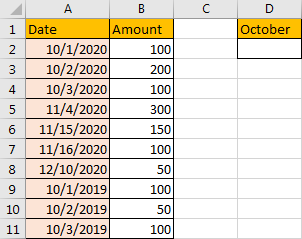Obviously, we have data for October in year 2020 and year 2019. We want to sum all items for October.

FORMULA:

In D2, enter the formula =SUMPRODUCT((MONTH(\$A\$2:\$A\$11)=10)*\$B\$2:\$B\$11).

HOW THIS FORMULA WORKS:

Inside of SUMPRODUCT function, we also use MONTH function in this instance. For MONTH function, the syntax is MONTH(serial_number). For example, if A1 is 01/01/2020, then MONTH(A1) returns 1. This function returns the month serial number. So, if we want to find out date in date range belongs to October, we need to use MONTH(\$A\$2:\$A\$11)=10 here, thus we can get an array as result.

Enter =(MONTH(A2:A11)=10) in D2 temporally. We get TRUE here, actually it only returns the first value of the array.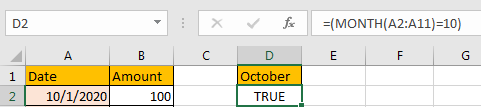Move focus in formula bar, then press F9 (on different version maybe Fn+F9). We can see the array is listed properly.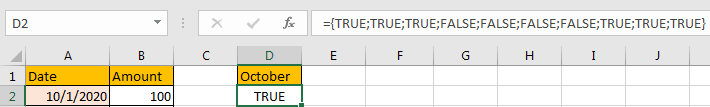Update formula to =–(MONTH(A2:A11)=10). Then TRUE or FALSE will convert to number properly.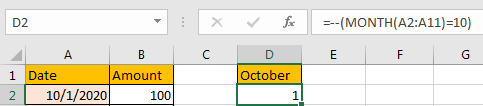Move focus in formula bar, then press F9.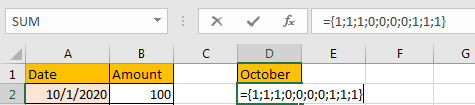For SUMPRODUCT function, the syntax is SUMPRODUCT(array1,[array2],[array3],…). After learning MONTH function, we all know that MONTH(\$A\$2:\$A\$11)=10 it will return an array. As SUMPRODUCT function can returns the sum of an array, and \$B\$2:\$B\$11 is another array for saving amount, and data in this range is also the match value for each date in A column. so here we can use =SUMPRODUCT ((MONTH(\$A\$2:\$A\$11)=10)*\$B\$2:\$B\$11) to sum data by month October properly. It equals to {1;1;1;0;0;0;0;1;1;1}*{100;200;100;300;150;100;50;100;50;100}, equivalent to =SUMPRODUCT({100;200;100;0;0;0;0;100;50;100}).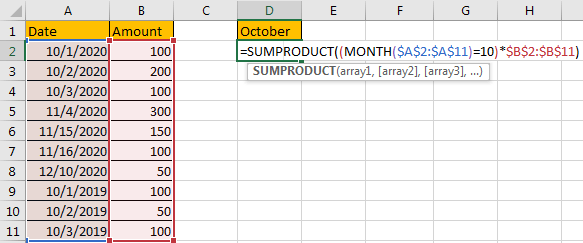RESULT:

Now we can sum data by month ignoring the year properly.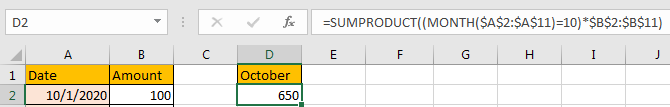### Related Functions

• Excel SUMPRODUCT function
The Excel SUMPRODUCT function multiplies corresponding components in the given one or more arrays or ranges, and returns the sum of those products. The syntax of the SUMPRODUCT function is as below:= SUMPRODUCT (array1,[array2],…)…
• Excel MONTH function
The Excel MONTH function returns the month of a date represented by a serial number. And the month is an integer number from 1 to 12. The syntax of the MONTH function is as below:=MONTH (serial_number)…